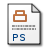# Perturbation theory for homogeneous polynomial eigenvalue problems

Tisseur, Françoise and Dedieu, Jean-Pierre (2003) Perturbation theory for homogeneous polynomial eigenvalue problems. Linear Algebra and its Applications, 385 (1). pp. 71-74. ISSN 0024-3795Postscript hpep.ps Download (329kB)

## Abstract

We consider polynomial eigenvalue problems P(A,alpha,beta)x=0 in which the matrix polynomial is homogeneous in the eigenvalue (alpha,beta)&unknown;C2. In this framework infinite eigenvalues are on the same footing as finite eigenvalues. We view the problem in projective spaces to avoid normalization of the eigenpairs. We show that a polynomial eigenvalue problem is well-posed when its eigenvalues are simple. We define the condition numbers of a simple eigenvalue (alpha,beta) and a corresponding eigenvector x and show that the distance to the nearest ill-posed problem is equal to the reciprocal of the condition number of the eigenvector x. We describe a bihomogeneous Newton method for the solution of the homogeneous polynomial eigenvalue problem (homogeneous PEP)

Item Type: Article Mathematical Subject Codes] 65F15; [Mathematical Subject Codes] 15A18; Polynomial eigenvalue problem; Matrix polynomial; Quadratic eigenvalue problem; Condition number MSC 2010, the AMS's Mathematics Subject Classification > 15 Linear and multilinear algebra; matrix theoryMSC 2010, the AMS's Mathematics Subject Classification > 65 Numerical analysis Ms Lucy van Russelt 05 Dec 2007 20 Oct 2017 14:12 http://eprints.maths.manchester.ac.uk/id/eprint/984View Item